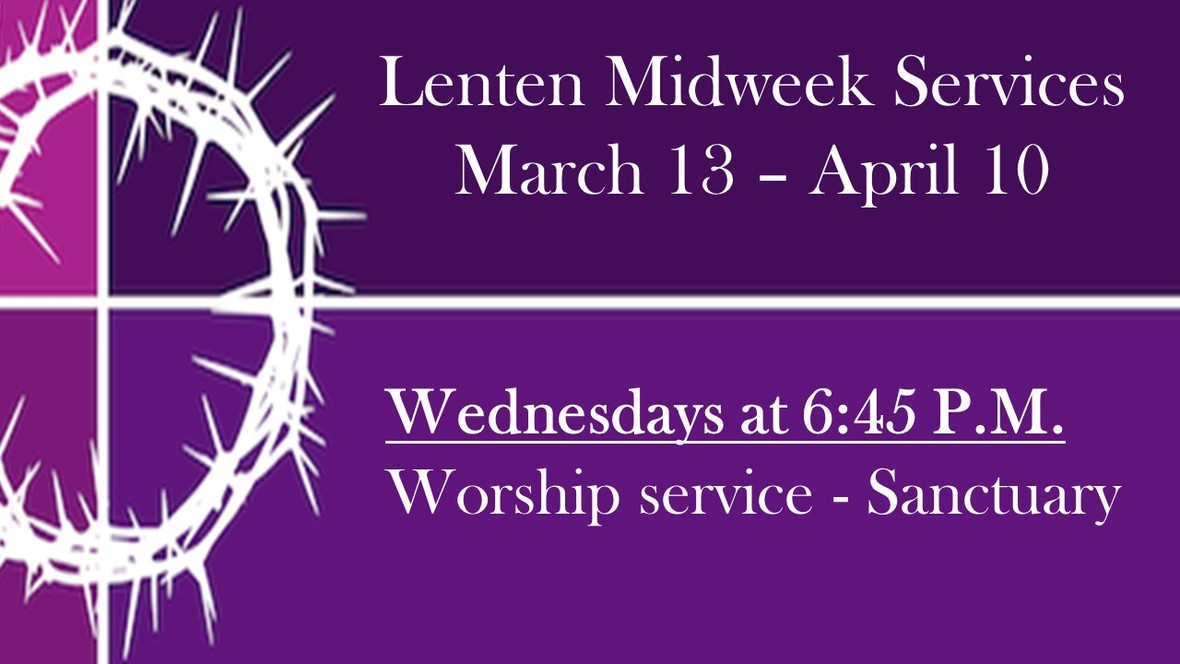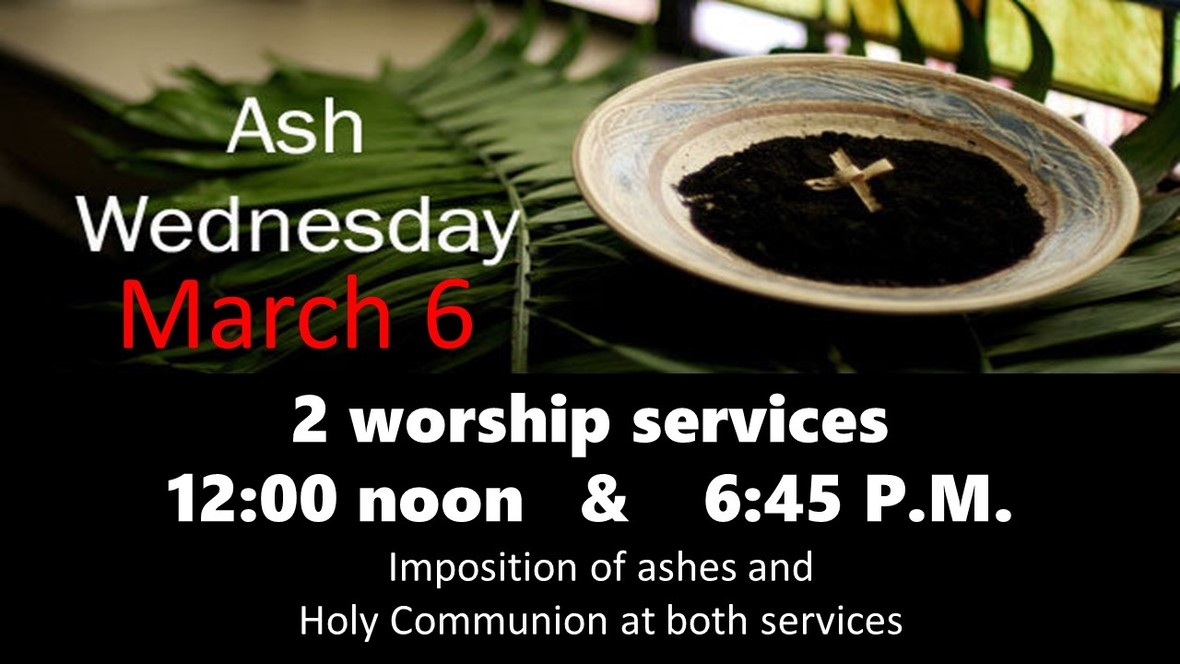Like   TweetPastoral ReflectionPastor Mark Hagen

Winter isn't the only season of length this time of year . . . read more.

 table div table+table+table+table div table{width:100%;padding:0}table div table+table+table+table div table img{width:96.23%;padding:0;float:none}table div table+table+table+table div table td{width:100%;padding:0 1.88% 18px}/* styles */This Week at St. Mark Feb.24 - March 3, 2019

 table div table+table+table+table+table+table div table{width:100%;padding:0}table div table+table+table+table+table+table div table img{width:96.23%;padding:0;float:none}table div table+table+table+table+table+table div table td{width:100%;padding:0 1.88% 18px}/* styles */Sunday, February 24

8:00 A.M. Worship
9:30 A.M. Worship
9:30 A.M. Installation of Council Members
9:30 A.M. Sunday School
11:00 A.M. Worship
12:00 P.M. High School Youth Event

Monday, February 25

9:00 A.M. Classic Chorale Rehearses
6:30 P.M. Emotions Anonymous

Tuesday, February 26

10:00 A.M. Staff Meeting
11:00 A.M. St. Mark Duplicate Bridge
7:00 P.M. Council Meeting

Wednesday, February 27

9:00 A.M. Women's Bible Study
9:00 A.M. Men's Bible Study
5:30 P.M. Good News Bearers
6:15 P.M. Bible Study
6:30 P.M. Confirmation
7:00 P.M. Voices of St. Mark

Thursday, February 28

7:30 A.M. Panera Book Study
9:00 A.M. Cherry Valley Bridge Group
1:00 P.M. Sew and Sews
6:30 P.M. Emotions Anonymous
6:30 P.M. Diakonia
7:00 P.M. Praise Band Rehearses

Sunday, March 3

8:00 A.M. Worship
9:30 A.M. Worship
9:30 A.M. Baptism of Charlie Mufich
9:30 A.M. Good News Bearers Perform
9:30 A.M. Sunday School
10:45 A.M. New Members Orientation
11:00 A.M. Worship

 table div table+table+table+table+table+table+table+table+table div table{width:100%;padding:0}table div table+table+table+table+table+table+table+table+table div table img{width:96.23%;padding:0;float:none}table div table+table+table+table+table+table+table+table+table div table td{width:100%;padding:0 1.88% 18px}/* styles */News and Events

 table div table+table+table+table+table+table+table+table+table+table+table div table{width:100%;padding:0}table div table+table+table+table+table+table+table+table+table+table+table div table img{width:96.23%;padding:0;float:none}table div table+table+table+table+table+table+table+table+table+table+table div table td{width:100%;padding:0 1.88% 18px}/* styles */Celebrating Bold Women
Have you ever thought about Mary as a bold woman? Our Advent memories of Mary’s song of justice have not waned so much that we can’t appreciate her boldness. During Epiphany, Mary persists, caring for the infant Jesus as visitors follow the star to find the Savior. How extraordinary is Mary’s faith, acceptance and willingness to serve! Her vision of God’s world foretells the bold landscape that her son Jesus ushers in.
Bold women are a part of our faith’s genealogy, including the woman at the well, the persistent widow, the women at the tomb, and those certain women who supported Jesus and the disciples. Within Women of the ELCA, we celebrate bold women on the fourth Sunday of February every year. On this day, congregations honor and remember bold women from their communities. These women may be members of the congregation, notable for acting boldly on their faith in some way. They may also be part of the larger community, notable for their boldness in helping to bring about healing and wholeness for the church, the society or the world. Join us this Sunday in worship as we honor bold women of faith.

 table div table+table+table+table+table+table+table+table+table+table+table+table+table div table{width:100%;padding:0}table div table+table+table+table+table+table+table+table+table+table+table+table+table div table img{width:96.23%;padding:0;float:none}table div table+table+table+table+table+table+table+table+table+table+table+table+table div table td{width:100%;padding:0 1.88% 18px}/* styles */New Member Orientation
The spring New Member Orientation dates are March 3 - 24 from 10:45-11:45 A.M. Please contact Pastor Chad McKenna at prmckenna@stmarklc.com if you are interested in becoming a member of St. Mark. We will receive new members on March 31 at the 9:30 A.M. service.

 table div table+table+table+table+table+table+table+table+table+table+table+table+table+table+table div table{width:100%;padding:0}table div table+table+table+table+table+table+table+table+table+table+table+table+table+table+table div table img{width:96.23%;padding:0;float:none}table div table+table+table+table+table+table+table+table+table+table+table+table+table+table+table div table td{width:100%;padding:0 1.88% 18px}/* styles */Are Winter Blues Getting You Down? Volunteering is sure to lift your spirit! Check out the latest volunteer opportunities in Rockford. See display in Fellowship Hall.

 table div table+table+table+table+table+table+table+table+table+table+table+table+table+table+table+table+table div table{width:100%;padding:0}table div table+table+table+table+table+table+table+table+table+table+table+table+table+table+table+table+table div table img{width:96.23%;padding:0;float:none}table div table+table+table+table+table+table+table+table+table+table+table+table+table+table+table+table+table div table td{width:100%;padding:0 1.88% 18px}/* styles *//* styles */ Mid-Week Lenten Service Join us on Wednesday evenings, starting March 13 at 6:45 P.M. in the sanctuary for worship. Our Lenten services will center on, "Five Gifts of Discipleship."table div table+table+table+table+table+table+table+table+table+table+table+table+table+table+table+table+table+table+table+table div table{width:100%;padding:0}table div table+table+table+table+table+table+table+table+table+table+table+table+table+table+table+table+table+table+table+table div table img{width:96.23%;padding:0;float:none}table div table+table+table+table+table+table+table+table+table+table+table+table+table+table+table+table+table+table+table+table div table td{width:100%;padding:0 1.88% 18px}/* styles */Lenten Devotionals
Free Lenten devotional booklets are available on the name tag tables in the narthex. This year’s devotions are entitled Thy Will Be Done by Ted Schroeder.

 table div table+table+table+table+table+table+table+table+table+table+table+table+table+table+table+table+table+table+table+table+table+table div table{width:100%;padding:0}table div table+table+table+table+table+table+table+table+table+table+table+table+table+table+table+table+table+table+table+table+table+table div table img{width:96.23%;padding:0;float:none}table div table+table+table+table+table+table+table+table+table+table+table+table+table+table+table+table+table+table+table+table+table+table div table td{width:100%;padding:0 1.88% 18px}/* styles */Miss a Sunday at St. Mark? Did you know that you can watch the sermons online? Click here to watch.

 table div table+table+table+table+table+table+table+table+table+table+table+table+table+table+table+table+table+table+table+table+table+table+table+table div table{width:100%;padding:0}table div table+table+table+table+table+table+table+table+table+table+table+table+table+table+table+table+table+table+table+table+table+table+table+table div table img{width:96.23%;padding:0;float:none}table div table+table+table+table+table+table+table+table+table+table+table+table+table+table+table+table+table+table+table+table+table+table+table+table div table td{width:100%;padding:0 1.88% 18px}/* styles */Attention All Singing Men
The Marksmen will be singing on Sunday, February 24 at the 8:00 and 9:30 A.M. services. This group is open to all men who like to sing and there is no ongoing commitment. We will meet at 7:00 A.M. in the choir room on February 24 to rehearse a simple anthem, based on the hymn "I Surrender All." Then we will sing it at the first two services that day. There is no obligation beyond that. Why not give it a try?

 table div table+table+table+table+table+table+table+table+table+table+table+table+table+table+table+table+table+table+table+table+table+table+table+table+table+table div table{width:100%;padding:0}table div table+table+table+table+table+table+table+table+table+table+table+table+table+table+table+table+table+table+table+table+table+table+table+table+table+table div table img{width:96.23%;padding:0;float:none}table div table+table+table+table+table+table+table+table+table+table+table+table+table+table+table+table+table+table+table+table+table+table+table+table+table+table div table td{width:100%;padding:0 1.88% 18px}/* styles */The 2019 altar flower chart is up in Fellowship Hall on the cabinets next to the coffee station on Sunday mornings. The arrangements will be \$35. This is a great way to honor or remember that special someone or that special anniversary.

 table div table+table+table+table+table+table+table+table+table+table+table+table+table+table+table+table+table+table+table+table+table+table+table+table+table+table+table+table div table{width:100%;padding:0}table div table+table+table+table+table+table+table+table+table+table+table+table+table+table+table+table+table+table+table+table+table+table+table+table+table+table+table+table div table img{width:96.23%;padding:0;float:none}table div table+table+table+table+table+table+table+table+table+table+table+table+table+table+table+table+table+table+table+table+table+table+table+table+table+table+table+table div table td{width:100%;padding:0 1.88% 18px}/* styles */Sign-up for an Ice Hogs Game
St. Mark is going to the game on Sunday, March 31, 4:00 P.M. game. Tickets are \$22. This game will honor the Greg Lindmark foundation. There will be autographs with Chris Chelios Join in on the fun! Please, sign-up in Fellowship Hall.

 table div table+table+table+table+table+table+table+table+table+table+table+table+table+table+table+table+table+table+table+table+table+table+table+table+table+table+table+table+table+table div table{width:100%;padding:0}table div table+table+table+table+table+table+table+table+table+table+table+table+table+table+table+table+table+table+table+table+table+table+table+table+table+table+table+table+table+table div table img{width:96.23%;padding:0;float:none}table div table+table+table+table+table+table+table+table+table+table+table+table+table+table+table+table+table+table+table+table+table+table+table+table+table+table+table+table+table+table div table td{width:100%;padding:0 1.88% 18px}/* styles */Congratulations to the following persons who were elected at the annual meeting, February 10. Council: - Maureen Hargrove, Christy Malenchik, Kraig Reichwald, Jeff Stovall. Audit Committee - Charles Kluzak, Endowment Committee - Chuck Schroder, Nominating Committee - Tayrn Fee, Synod Delegates - Don and Pat Coffman, Sharon Erickson.
The council will be installed this Sunday at the 9:30 service. We thank you for your commitment to St. Mark.

 table div table+table+table+table+table+table+table+table+table+table+table+table+table+table+table+table+table+table+table+table+table+table+table+table+table+table+table+table+table+table+table+table div table{width:100%;padding:0}table div table+table+table+table+table+table+table+table+table+table+table+table+table+table+table+table+table+table+table+table+table+table+table+table+table+table+table+table+table+table+table+table div table img{width:96.23%;padding:0;float:none}table div table+table+table+table+table+table+table+table+table+table+table+table+table+table+table+table+table+table+table+table+table+table+table+table+table+table+table+table+table+table+table+table div table td{width:100%;padding:0 1.88% 18px}/* styles */First Tuesday Book Club
The book club will meet at 1:00 P.M. on March 7 and will be hosted by Judy Penticoff. Please email her at teachem4@comcast.net and let her know if you will attend, or, leave a message at 815 985-3427. Please join us! We’ll read and discuss Before We Were Yours by Lucy Wingate.
Looking ahead our April book is Need to Know by Karen Carpenter.

 table div table+table+table+table+table+table+table+table+table+table+table+table+table+table+table+table+table+table+table+table+table+table+table+table+table+table+table+table+table+table+table+table+table+table div table{width:100%;padding:0}table div table+table+table+table+table+table+table+table+table+table+table+table+table+table+table+table+table+table+table+table+table+table+table+table+table+table+table+table+table+table+table+table+table+table div table img{width:96.23%;padding:0;float:none}table div table+table+table+table+table+table+table+table+table+table+table+table+table+table+table+table+table+table+table+table+table+table+table+table+table+table+table+table+table+table+table+table+table+table div table td{width:100%;padding:0 1.88% 18px}/* styles */Youth MinistrySt. Mark High School Youth High Adventure Trip
Apostle Islands High Adventure Trip June 23-28th 2019. Cost is \$500 and includes all food, lodging, kayak, etc. Please see Melinda Alekna if you have questions and/or want to sign up. Check out the web site www.Adv-Vac.com.

High School youth meet at St. Mark on Sunday, February 24 at noon. We will go to lunch and learn about upcoming youth opportunities. Bring money for your lunch.

Second Sunday is for high school youth and their friends. Join us at Katie’s Cup at 7:00 P.M.on March 10. All high school youth and friends are invited. Fun, friends, games, youth led worship and music!

 table div table+table+table+table+table+table+table+table+table+table+table+table+table+table+table+table+table+table+table+table+table+table+table+table+table+table+table+table+table+table+table+table+table+table+table+table+table div table{width:100%;padding:0}table div table+table+table+table+table+table+table+table+table+table+table+table+table+table+table+table+table+table+table+table+table+table+table+table+table+table+table+table+table+table+table+table+table+table+table+table+table div table img{width:96.23%;padding:0;float:none}table div table+table+table+table+table+table+table+table+table+table+table+table+table+table+table+table+table+table+table+table+table+table+table+table+table+table+table+table+table+table+table+table+table+table+table+table+table div table td{width:100%;padding:0 1.88% 18px}/* styles */table div table+table+table+table+table+table+table+table+table+table+table+table+table+table+table+table+table+table+table+table+table+table+table+table+table+table+table+table+table+table+table+table+table+table+table+table+table+table+table div table{width:100%;padding:0}table div table+table+table+table+table+table+table+table+table+table+table+table+table+table+table+table+table+table+table+table+table+table+table+table+table+table+table+table+table+table+table+table+table+table+table+table+table+table+table div table img{width:96.23%;padding:0;float:none}table div table+table+table+table+table+table+table+table+table+table+table+table+table+table+table+table+table+table+table+table+table+table+table+table+table+table+table+table+table+table+table+table+table+table+table+table+table+table+table div table td{width:100%;padding:0 1.88% 18px}/* styles */Outreach MinistryThe Outreach Committee thanks our St. Mark members for sewing the fleece throw blankets together to make warm quilts for the kids at Rock House Kids. The Sew and Sews finished 20 more blankets so it was quite a collection delivered to Rock House. We are very grateful for the help with this project.

 table div table+table+table+table+table+table+table+table+table+table+table+table+table+table+table+table+table+table+table+table+table+table+table+table+table+table+table+table+table+table+table+table+table+table+table+table+table+table+table+table+table+table div table{width:100%;padding:0}table div table+table+table+table+table+table+table+table+table+table+table+table+table+table+table+table+table+table+table+table+table+table+table+table+table+table+table+table+table+table+table+table+table+table+table+table+table+table+table+table+table+table div table img{width:96.23%;padding:0;float:none}table div table+table+table+table+table+table+table+table+table+table+table+table+table+table+table+table+table+table+table+table+table+table+table+table+table+table+table+table+table+table+table+table+table+table+table+table+table+table+table+table+table+table div table td{width:100%;padding:0 1.88% 18px}/* styles */table div table+table+table+table+table+table+table+table+table+table+table+table+table+table+table+table+table+table+table+table+table+table+table+table+table+table+table+table+table+table+table+table+table+table+table+table+table+table+table+table+table+table+table+table div table{width:100%;padding:0}table div table+table+table+table+table+table+table+table+table+table+table+table+table+table+table+table+table+table+table+table+table+table+table+table+table+table+table+table+table+table+table+table+table+table+table+table+table+table+table+table+table+table+table+table div table img{width:96.23%;padding:0;float:none}table div table+table+table+table+table+table+table+table+table+table+table+table+table+table+table+table+table+table+table+table+table+table+table+table+table+table+table+table+table+table+table+table+table+table+table+table+table+table+table+table+table+table+table+table div table td{width:100%;padding:0 1.88% 18px}/* styles */St. Mark Concerttable div table+table+table+table+table+table+table+table+table+table+table+table+table+table+table+table+table+table+table+table+table+table+table+table+table+table+table+table+table+table+table+table+table+table+table+table+table+table+table+table+table+table+table+table+table+table+table div table{width:100%;padding:0}table div table+table+table+table+table+table+table+table+table+table+table+table+table+table+table+table+table+table+table+table+table+table+table+table+table+table+table+table+table+table+table+table+table+table+table+table+table+table+table+table+table+table+table+table+table+table+table div table img{width:96.23%;padding:0;float:none}table div table+table+table+table+table+table+table+table+table+table+table+table+table+table+table+table+table+table+table+table+table+table+table+table+table+table+table+table+table+table+table+table+table+table+table+table+table+table+table+table+table+table+table+table+table+table+table div table td{width:100%;padding:0 1.88% 18px}/* styles */Our St. Mark members:

Inara McGroarty, Marsha Carlson, Steve Carter, Dick Zimmanck, David Young, Russ Oellig, Linda Swanson, Pam Cottrill, Keith Haggestad, Naomi Hagen.

Family and friends of St. Mark members:

Mary Graham, Barbara, Heckart, Tom Wolfe, Kim Menke, Sarah Adams, James Elder, Norma Comstock, Butch Cotter, Scott Jones, Ethan Whitney, Carolyn King, Tom Severson, Katie Butera, Kate Nickell, Ashton Henley, Nathan Gyllin.

Those serving in the military and their families:

Bailey Panjkovich, Xander Gehrke, Jim Tammen, Brandon Ballenger, Taylor Eversole, Dean Barron.

 table div table+table+table+table+table+table+table+table+table+table+table+table+table+table+table+table+table+table+table+table+table+table+table+table+table+table+table+table+table+table+table+table+table+table+table+table+table+table+table+table+table+table+table+table+table+table+table+table+table+table div table{width:100%;padding:0}table div table+table+table+table+table+table+table+table+table+table+table+table+table+table+table+table+table+table+table+table+table+table+table+table+table+table+table+table+table+table+table+table+table+table+table+table+table+table+table+table+table+table+table+table+table+table+table+table+table+table div table img{width:96.23%;padding:0;float:none}table div table+table+table+table+table+table+table+table+table+table+table+table+table+table+table+table+table+table+table+table+table+table+table+table+table+table+table+table+table+table+table+table+table+table+table+table+table+table+table+table+table+table+table+table+table+table+table+table+table+table div table td{width:100%;padding:0 1.88% 18px}/* styles *//* styles */ Office Hours Monday - Friday, 8:00 A.M. - 4:00 P.M. Telephone: 815-398-3557 Publications St. Mark Memos, the weekly e-newsletter, is distributed each Thursday. The deadline for submission is Monday by 4:00 P.M. Please click to send submissions. Sermons Online Miss a Sunday at St. Mark? Please visit our website and watch the sermons online. Please click to watch.
 /* styles */